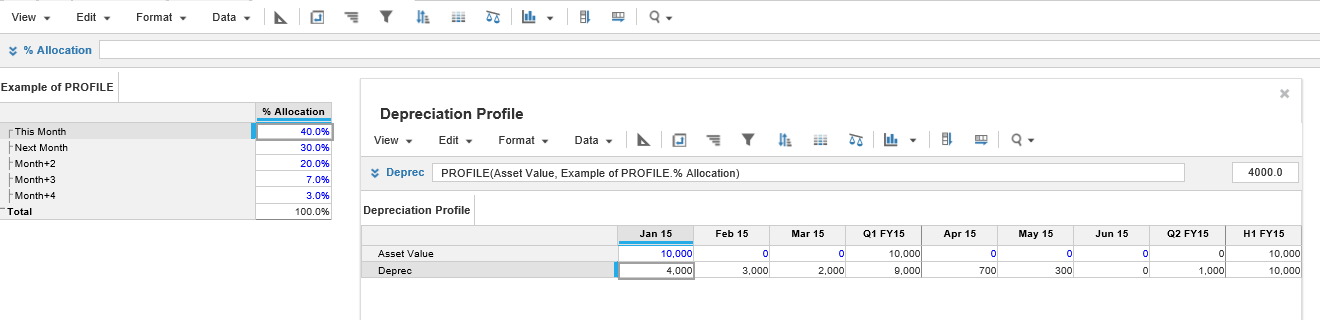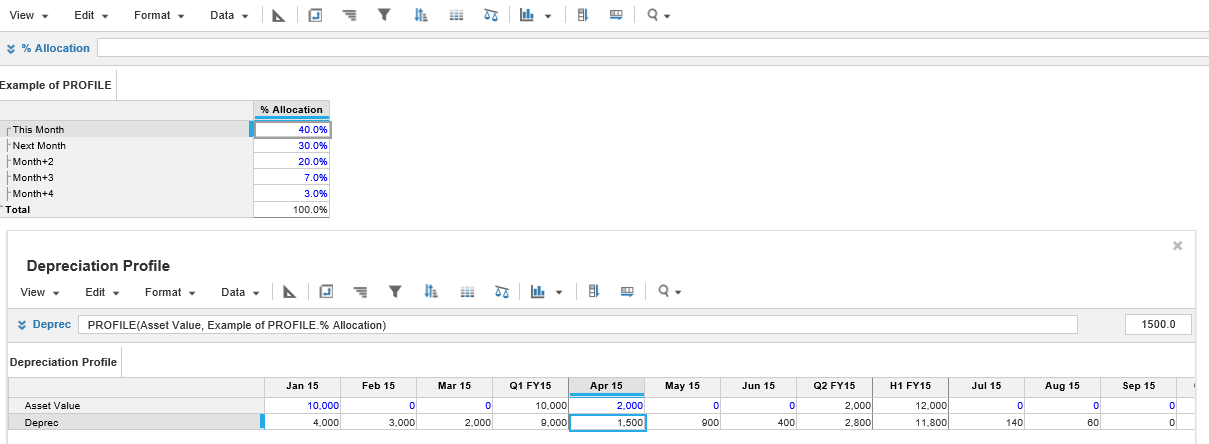1. Calculation functions
2. All Functions
3. Time and Date Functions
4. PROFILE

This function allocates a value (x) over future periods to spread data values forward over time by applying the specified profile. The future periods are set up on a table containing % current period, % next period, % pd+2 etc.

## Syntax

PROFILE(x, y)

where:

• x: Value to be distributed
• y: % Allocation

## Format

Input Format Output Format

x: Number

y: Number that contains % Allocation

Number

## Arguments

The function uses the following arguments:

• x: Number: Numeric line item, property, or expression
• y: Number: Numeric line item, property, or expression

## Constraints

The function has the following constraints:

• The first dimension of the profile must be the time-offsets (this month, next month...).
• The time range providing arguments to a line item must match the time range for the result line item. Any formula that conflicts with this rule will be rejected. For more information, see Search page.
• A non-time series function, used as a sub-expression of this function, must use the same time range as the line item to which the formula is applied. Combining time series functions and non-time series functions with a time range could return an unexpected result or an error message. For more information, see Time Ranges and Time Series Functions page.

## Example

In this example, we have a simple Depreciation Profile module with an Asset Value row.

The depreciation profile is held in the Example of PROFILE Module.The depreciation figure is detailed on the Deprec row using the formula:

Deprec = PROFILE(Asset Value, Example of PROFILE.% Allocation)

The Deprec row takes the Asset Value and writes it off using the settings in the profile.

If an additional amount is added in the Asset Value row, the calculation is applied and the overlapping values are totaled.